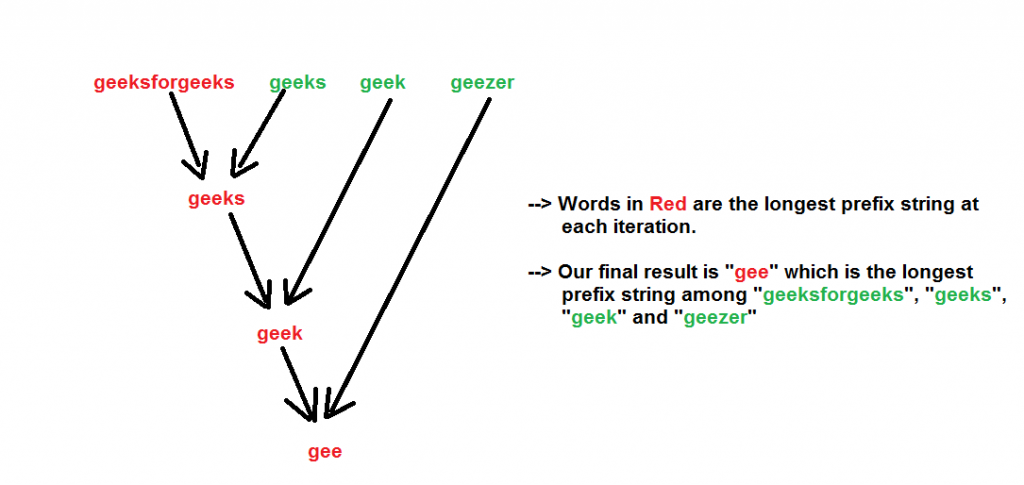GeeksforGeeks App
Open AppBrowser
Continue

# Python Program To Find Longest Common Prefix Using Word By Word Matching

Given a set of strings, find the longest common prefix.
Examples:

```Input  : {“geeksforgeeks”, “geeks”, “geek”, “geezer”}
Output : "gee"

Input  : {"apple", "ape", "april"}
Output : "ap"```

We start with an example. Suppose there are two strings- “geeksforgeeks” and “geeks”. What is the longest common prefix in both of them? It is “geeks”.
Now let us introduce another word “geek”. So now what is the longest common prefix in these three words ? It is “geek”
We can see that the longest common prefix holds the associative property, i.e-

```LCP(string1, string2, string3)
= LCP (LCP (string1, string2), string3)

Like here

LCP (“geeksforgeeks”, “geeks”, “geek”)
=  LCP (LCP (“geeksforgeeks”, “geeks”), “geek”)
=  LCP (“geeks”, “geek”) = “geek”```

So we can make use of the above associative property to find the LCP of the given strings. We one by one calculate the LCP of each of the given string with the LCP so far. The final result will be our longest common prefix of all the strings.
Note that it is possible that the given strings have no common prefix. This happens when the first character of all the strings are not same.
We show the algorithm with the input strings- “geeksforgeeks”, “geeks”, “geek”, “geezer” by the below figure.Below is the implementation of above approach:

## Python3

 `# Python3 Program to find the longest``# common prefix` `# A Utility Function to find the common``# prefix between strings- str1 and str2``def` `commonPrefixUtil(str1, str2):``    ``result ``=` `"";``    ``n1 ``=` `len``(str1)``    ``n2 ``=` `len``(str2)` `    ``# Compare str1 and str2``    ``i ``=` `0``    ``j ``=` `0` `    ``while` `i <``=` `n1 ``-` `1` `and` `j <``=` `n2 ``-` `1``:   ``        ``if` `(str1[i] !``=` `str2[j]):``            ``break``            ` `        ``result ``+``=` `str1[i]``        ``i ``+``=` `1``        ``j ``+``=` `1` `    ``return` `(result)` `# A Function that returns the longest``# common prefix from the array of strings``def` `commonPrefix (arr, n):``    ``prefix ``=` `arr[``0``]` `    ``for` `i ``in` `range` `(``1``, n):``        ``prefix ``=` `commonPrefixUtil(prefix,``                                  ``arr[i])``    ``return` `(prefix)` `# Driver Code``if` `__name__ ``=``=``"__main__"``:``    ``arr ``=` `[``"geeksforgeeks"``, ``"geeks"``,``           ``"geek"``, ``"geezer"``]``    ``n ``=` `len``(arr)``    ``ans ``=` `commonPrefix(arr, n)` `    ``if` `(``len``(ans)):``        ``print` `(``"The longest common prefix is -"``,``                ``ans);``    ``else``:``        ``print``(``"There is no common prefix"``)` `# This code is contributed by ita_c`

Output

`The longest common prefix is - gee`

Time Complexity : Since we are iterating through all the strings and for each string we are iterating though each characters, so we can say that the time complexity is O(N M) where,

```N = Number of strings
M = Length of the largest string string ```

Auxiliary Space : To store the longest prefix string we are allocating space which is O(M). Please refer complete article on Longest Common Prefix using Word by Word Matching for more details!

Method #2:

In this approach, the first word is taken as the longest common prefix, and then each subsequent word is compared to the prefix character by character. The prefix is updated as needed so that it only includes characters that are common to all the words. This process continues until all the words have been compared and the longest common prefix has been found.

Approach:

1. Define the function find_longest_common_prefix that takes a list of words as input.

2. Initialize the variable longest_common_prefix to the first word in the list of words.

3. Loop through each word in the list of words, starting from the second word.

4. Loop through each character in the current longest common prefix.

5. If the current character is not the same as the character in the same position in the current word, update the longest_common_prefix variable to the substring of the longest common prefix up to the index of the current character and break out of the loop.

6. If the loop completes without breaking, the longest_common_prefix variable will be equal to the shortest word in the list of words.

7. Return the longest_common_prefix variable.

## Python3

 `def` `find_longest_common_prefix(words):``    ``# Initialize the longest common prefix to the first word``    ``longest_common_prefix ``=` `words[``0``]` `    ``# Loop through each word in the list of words``    ``for` `word ``in` `words[``1``:]:``        ``# Loop through each character in the current longest common prefix``        ``for` `i ``in` `range``(``len``(longest_common_prefix)):``            ``# If the current character is not the same as the character in the same position in the current word``            ``if` `i >``=` `len``(word) ``or` `longest_common_prefix[i] !``=` `word[i]:``                ``# Update the longest common prefix and break out of the loop``                ``longest_common_prefix ``=` `longest_common_prefix[:i]``                ``break` `    ``# Return the longest common prefix``    ``return` `longest_common_prefix``words ``=` `[``"geeksforgeeks"``, ``"geeks"``, ``"geek"``, ``"geezer"``]``longest_common_prefix ``=` `find_longest_common_prefix(words)``print``(``"The longest common prefix is:"``, longest_common_prefix)`

Output

`The longest common prefix is: gee`

Overall, the function has a time complexity of O(mn)
Auxiliary space: O(1), where m is the length of the longest word and n is the number of words in the list.

My Personal Notes arrow_drop_up